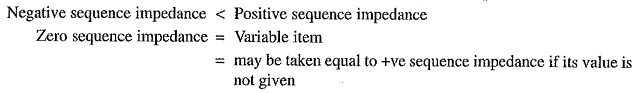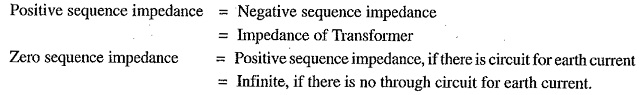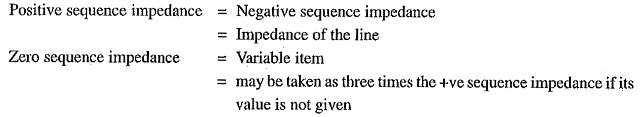## Sequence Impedance of Power System:

Each element of power system will offer impedance to different phase sequence components of current which may not be the same. For example, the impedance which any piece of equipment offers to positive sequence current will not necessarily be the same as offered to negative sequence current or zero sequence current. Therefore, in unsymmetrical fault calculations, each piece of equipment will have three values of impedance one corresponding to each Sequence Impedance of Power System current viz.

• Positive sequence impedance (Z1)
• Negative sequence impedance (Z2)
• Zero sequence impedance (Z0)

The impedance offered by an equipment or circuit to positive sequence current is called positive Sequence Impedance of Power System and is represented by Z1. Similarly, impedances offered by any circuit or equip­ment to negative and zero sequence currents are respectively called negative sequence impedance (Z2) and zero sequence impedance (Z0).

The following points may be noted :

1. In a 3-phase balanced system, each piece of equipment or circuit offers oply one impedance–the one offered to positive or normal sequence current. This is ekpected because of the absence of negative and zero sequence currents in the 3-phase balanced system.
2. In a 3-phase unbalanced system, each piece of equipment or circuit will have three values of impedance positive sequence impedance, negative sequence impedance and zero Sequence Impedance of Power System.
3. The positive and negative sequence impedances of linear, symmetrical and static circuits (e.g. transmission lines, cables, transformers and static loads) are equal and are the same as those used in the analysis of balanced conditions. This is due to the fact that impedance of such circuits is independent of the phase order, provided the applied voltages are balanced. It may be noted that positive and negative Sequence Impedance of Power System of rotating machines (e.g. synchronous and induction motors) are normally different.
4. The zero sequence impedance depends upon the path taken by the zero sequence current. As this path is generally different from the path taken by the positive and negative sequence currents, therefore, zero sequence impedance is usually different from positive or negative sequence impedance.

### Sequence Impedances of Power System Elements:

The concept of impedances of various elements of power system (e.g. generators, transformers, transmission lines etc.) to positive, negative and zero sequence currents is of considerable importance in determining the fault currents in a 3-phase unbalanced system. A complete consideration of this topic does not fall within the scope of this book, but a short preliminary explanation may be of interest here. The following three main pieces of equipment will be considered :

• Synchronous generators
• Transformers
• Transmission lines

(i) Synchronous generators: The positive, negative and zero sequence impedances of rotating machines are generally different. The positive sequence impedance of a synchronous generator is equal to the synchronous impedance of the machine. The negative sequence impedance is much less than the positive Sequence Impedance of Power System. The zero sequence impedance is a variable item and if its value is not given, it may be assumed to be equal to the positive sequence impedance. In short :It may be worthwhile to mention here that any impedance Ze in the earth connection of a star-connected system has the effect to introduce an impedance of 3 Ze per phase. It is because the three equal zero-sequence currents, being in phase, do not sum to zero at the star point, but they flow back along the neutral earth connection.

(ii) Transformers: Since transformers have the same impedance with reversed phase rotation, their positive and negative sequence impedances are equal; this value being equal to the impedance of the transformer. However, the zero sequence impedance depends upon earth connection. If there is a through circuit for earth current, zero sequence impedance will be equal to positive sequence impedance otherwise it will be infinite. In short,(iii) Transmission lines: The positive sequence and negative sequence impedance of a line are the same; this value being equal to the normal impedance of the line. This is expected because the phase rotation of the currents does not make any difference in the constants of the line. However, the zero sequence impedance is usually much greater than the positive or negative Sequence Impedance of Power System. In short :